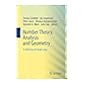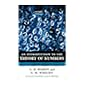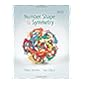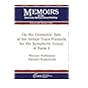Normal view MARC view ISBD view

# Number theory revealed : a masterclass / Andrew Granville.

Material type:TextPublisher: Providence, Rhode Island : American Mathematical Society, Description: xxviii, 587 pages; USD 99.00 26 cms.Content type: text Media type: unmediated Carrier type: volumeISBN: 9781470441586.DDC classification: 512.7 Other classification: 11-01 | 11A55 | 11B30 | 11B39 | 11D04 | 11D09 | 11D25 | 11N05 | 11N25
Contents:
Gauss's Disquisitiones Arithmeticae -- Preliminary chapter on induction -- The Euclidean algorithm -- Congruences -- The basic algebra of number theory -- Multiplicative functions -- The distribution of prime numbers -- Diophantine problems -- Power residues -- Quadratic residues -- Quadratic equations -- Square roots and factoring -- Rational approximations to real numbers -- Binary quadratic forms -- The anatomy of integers -- Counting integral and rational points on curves, mod p -- Combinatorial number theory -- The p-adic numbers -- Rational points on elliptic curves.
List(s) this item appears in: 2020-03-06
Item type Current location Call number Status Date due Barcode Item holds
Book Chennai Mathematical Institute
General Stacks
512.7 GRA (Browse shelf) Available 10791
Total holds: 0
##### Browsing Chennai Mathematical Institute Shelves , Shelving location: General Stacks Close shelf browser
 No cover image available No cover image availableNo cover image available No cover image available512.7 GOL Automorphic representations and L-functions for the general linear group /Vol II 512.7 GOL Number theory, analysis and geometry : in memory of Serge Lang / 512.7 GOL Automorphic representations and L-functions for the general linear group /Vol. I 512.7 GRA Number theory revealed : a masterclass / 512.7 HAR An introduction to the theory of numbers / 512.7 HER Number, shape, and symmetry : an introduction to number theory, geometry, and group theory / 512.7 HOF On the geometric side of the Arthur trace formula for the symplectic group of rank 2 /

Includes bibliographical references and index.

Gauss's Disquisitiones Arithmeticae -- Preliminary chapter on induction -- The Euclidean algorithm -- Congruences -- The basic algebra of number theory -- Multiplicative functions -- The distribution of prime numbers -- Diophantine problems -- Power residues -- Quadratic residues -- Quadratic equations -- Square roots and factoring -- Rational approximations to real numbers -- Binary quadratic forms -- The anatomy of integers -- Counting integral and rational points on curves, mod p -- Combinatorial number theory -- The p-adic numbers -- Rational points on elliptic curves.